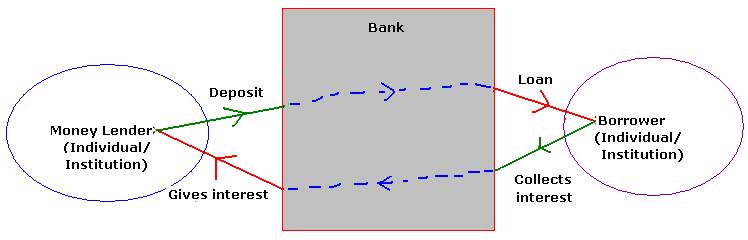4.5 Simple Interest:

The money given by a depositor to Bank or the money given by bank to a borrower is called ‘PRINCIPAL’ Amount.   The duration for which   money given by depositor to the Bank or the duration for which money is taken on loan from bank is called ‘PERIOD or TERM’ The interest one gets from bank or the interest charged by a Bank on a loan is normally expressed as a % for an year. Since Banks  also have to make profit after paying for  expenses ( rent for the building, salary to its employees printing of stationary, electricity and other expenses). Bank’s major income comes from interest charged on loans.  They also pay interest to depositors.  Thus, in order that Banks are profitable they charge more interest on loans (around 5% more) when compared to interest given on deposits.4.5 Example 1:Ram has deposited 5000Rs in State Bank of India for 6 years at 8% interest.( This means that the Bank has  collected an initial deposit amount of Rs 5000  on the condition that   this amount will be with   the bank for a period of 6 years. For having given the money to bank, the bank agrees to pay him interest at 8% per year for 6 long years) How much interest will he get in one year and  in six years?

What does 8% interest mean to Ram? (The bank agrees to pay 8 Rupees for every 100 Rupees every year)

It is given that for Rs. 100 he gets 8 Rs per year (8% interest)

For Rs. 5000 he gets 8*5000/100 = 400Rs per year

Since he has deposited Rs.5000, he should get Rs.400 every year for 6 years

In all he will get Rs.2400 (=400*6) as interest in 6 years from Bank

He will also get back his principal amount of 5000Rs at the end of 6 years

In banking terms this method of arriving interest is called ‘Simple Interest’

If

P = Principal amount (The sum borrowed or the sum lent)

N = Period (Term) of Deposit/loan  in years

R = Rate of Interest (The interest amount on every Rs 100 for one year)

I = The interest money paid by borrower to the money lender or to the bank for use of money (P) borrowed

A =The amount paid/payable at the end of period then(A = P+I )

For simple Interest calculation we use the formula:

Simple Interest (SI)= (P*N*R)/100

Let us verify correctness of   our interest calculation in the above example using the above formula

Verification:

P = 5000

N= 6years

R = 8%

Substituting these values in the above formula we get

Interest = (P*N*R)/100

= (5000*6*8)/100 = 50*6*008 =2400 which is what we arrived in the example

Since A = P+I and I = P*N*R/100

A = P+ (P*N*R)/100 = P{1+ (N*R)/100}

Let us take the case of borrower.

4.5 Problem 2: Mr. Raj borrows from State Bank of India a loan amount of   Rs. 1,50,000 for 7 years at the rate of 12% interest, for starting a business. Find out how much interest he has to pay in all in 7 years to the Bank.  Also find out what amount he has to give to the bank at the time of return of loan (Assume that he pays the interest on loan at the end along with loan amount)

Solution :

In this case we have

P = 150000

R= 12

N =7

Substituting these values in the formula we get

Total interest = 150000*7*(12/100) = 1500*7*12 = 126000

Since he also pays principal loan amount at the end,  his total payment to bank will be Rs 2,76,000(=1,50,000as principal +1,26,000 as Interest)

4.5 Problem 3: A sum of money amounts to Rs 3,360 at 14% Simple interest in 3 years. Find the interest on the same sum for 3 1/2 months at 6%

Solution :

In this case we have

A = 3360, R =14 and N=3

But A = P{1+ (N*R)/100}

3360 = P{1+ (3*14)/100} = p*142/100P = 3360*100/142 = 2366

R = 6 and N = 3 1/2 months= 3.5/12 years

SI = (P*N*R)/100= {2366*(3.5/12)*6/100} = Rs. 41.41

4.5 Problem 4:  Three sisters are 5, 10 and 15 years old. Their father leaves Rs80,250 in a bank; paying 5% simple interest. If each gets the same amount at the age of 20, find their shares at the time of father’s death.

Solution :

Since each of the sister  get the amount at the age of 20 years. Let the sister’s share be P1,P2 and P3 respectively

First sister’s share (P1) is held in the bank for 15 years

Second sister’s share (P2) is held in the bank for 10 years

Third sister’s share (P3) is held in the bank for 5 years

The amount got by first sister after 15 years

= P1{1+ (N*R)/100}= P1{1+ (15*5)/100} =1.75 P1

The amount got by second sister after 10 years

= P2 {1+ (N*R)/100}= P2 {1+ (10*5)/100} =1.5 P2

The amount got by third sister after 5 years

= P3{1+ (N*R)/100}= P3{1+ (5*5)/100} =1.25 P3

Since it is given that the amount they get at the age of 20 years is same

1.75 P1=1.5 P2=1.25 P3

On simplification we get

7P1=6P2=5P3P2 = 7/6P1

and P3 = 7/5P1

But it is given that P1+P2+P3 = 80250P1+7/6P1+7/5P1= 80250

I.e. (30+35+42)/30 P1= 80250

I.e. P1= 80250*30/107 =  22500

By substituting this value we find that

P2 = 7/6P1= 26250

P3 = 7/5P1= 31500

The sisters share at the time of their father’s death is Rs 22,500, Rs26,250 and Rs,31,500.

Verify using the formula that these deposits fetch same amount when the sisters attain the age of 20 years.

4.5 Summary of learning

 No Points to remember 1 Simple Interest (SI)= P*N*(R/100)  Where P = Principal Amount N = Period (Term) of Deposit: R = Rate of Interest